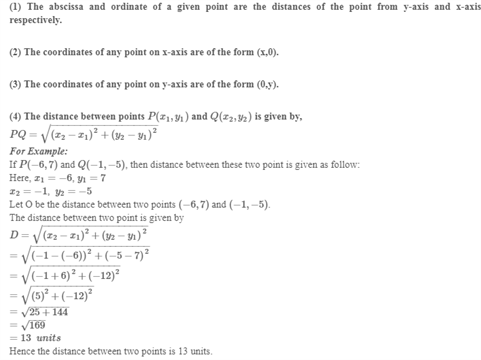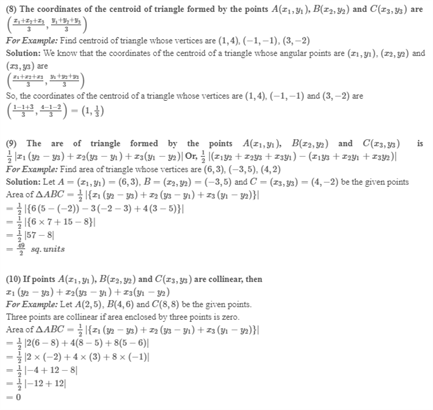# Revision Notes For CBSE Class 10 Maths Chapter 7 Coordinate Geometry

Chapter 7 given in class 10 maths book is an interesting chapter as it focuses more on applying math concepts to real word problems. To give you a brief idea, students have to learn about formulas like distance and section formula where they will need to understand its application in finding the distance between the two points whose coordinates are mentioned. The chapter also talks about other topics like the area of a triangle and contains a lot of exercises. Notably, it is important for students to be thorough with the concepts and then only they can master this chapter completely. We are providing free CBSE revision notes for class 10 maths chapter 7 – coordinate geometry here to help students get a good grasp of the important concepts and solve math problems without any difficulty. Students can make use of these notes to make the most out of their study time and perform well in the exams.

Class 10 maths chapter 6 CBSE revision notes PDF is provided below.Access CBSE Class 10 Maths Sample Papers Here.

Access NCERT Class 10 Maths Book Here.